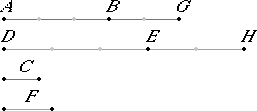# Proposition 2

If a first magnitude is the same multiple of a second that a third is of a fourth, and a fifth also is the same multiple of the second that a sixth is of the fourth, then the sum of the first and fifth also is the same multiple of the second that the sum of the third and sixth is of the fourth.
V.Def.2

Let a first magnitude AB be the same multiple of a second C that a third DE is of a fourth F, and let a fifth BG be the same multiple of the second C that a sixth EH is of the fourth F.

I say that the sum AG of the first and fifth is the same multiple of the second, C, that the sum DH of the third and sixth is of the fourth, F.

Since AB is the same multiple of C that DE is of F, therefore there are as many magnitudes in AB equal to C as there are in DE equal to F.For the same reason there are as many magnitudes in BG equal to C as there are in EH equal to F. Therefore, there are as many magnitudes in the whole AG equal to C as there are in the whole DH equal to F.

Therefore, AG is the same multiple of C that DH is of F.

Therefore the sum AG of the first and fifth is the same multiple of the second, C, that the sum DH of the third and sixth is of the fourth, F.

Therefore, if a first magnitude is the same multiple of a second that a third is of a fourth, and a fifth also is the same multiple of the second that a sixth is of the fourth, then the sum of the first and fifth also is the same multiple of the second that the sum of the third and sixth is of the fourth.

Q.E.D.

## Guide

This proposition simply states that sums of equimultiples are equimultiples, that is, if mc and mf are equimultiples of c and f, and nc and nf are also equimultiples of c and f, then the sums mc + nc and mf + nf are also equimultiples of c and f. The proof depends on a form of distributivity, namely, that multiplication by magnitudes distributes over addition of numbers.

(m + n)c = mc + nc.

Note that the magnitudes don’t all have to be of the same kind. Different colors are used in the figures here to indicate different kinds of magnitudes.

#### Use of this proposition

Proposition V.2 is used in the proofs of three other propositions, namely, V.3, V.6, and V.17.